#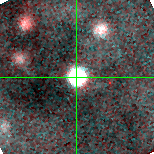M33C-19725 (J013339.52+304540.5)

## Plots of Brightness and Color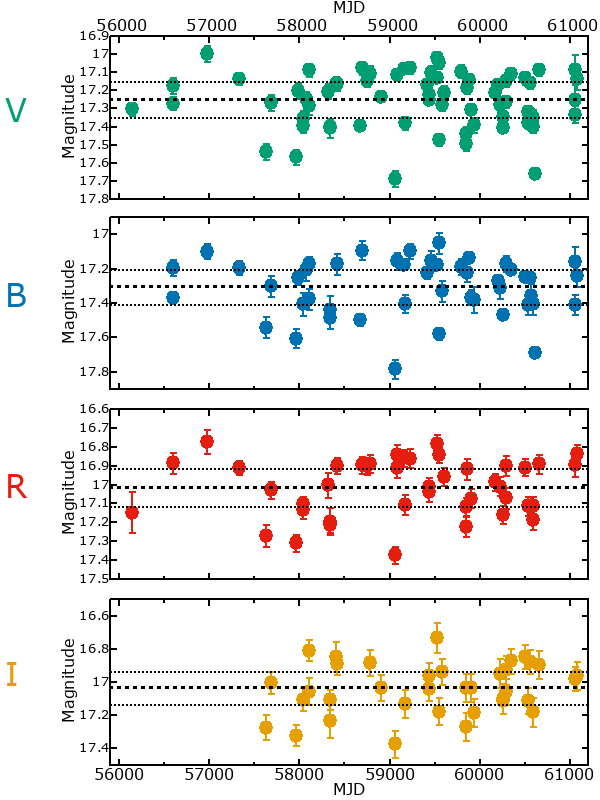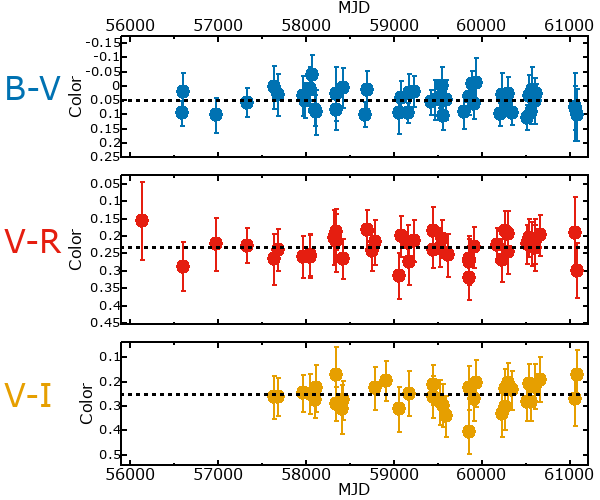## Variability

It is very likely that this star is variable.

### Correlation factors between bands

If the brightness variation is true variability then there should be a correlation between brightness changes recorded in different filter bands. The following table are the R-squared linear correlation factors calculated for the pairs of bands. The number in parentheses next to the R-squared value is the number of elements used to calculate the correlation factor. The number in square brackets next to the band is the range of magnitudes measured.

V [0.69 mag]B [0.71 mag]R [0.59 mag]
B [0.69 mag]0.96 (22)
R [0.59 mag]0.96 (21)0.93 (16)
I [0.59 mag]0.95 (14)0.92 (10)0.98 (10)

### Magnitude-Magnitude Plots

The plots below are provided to check the values in the table above and also to show any non-linear correlations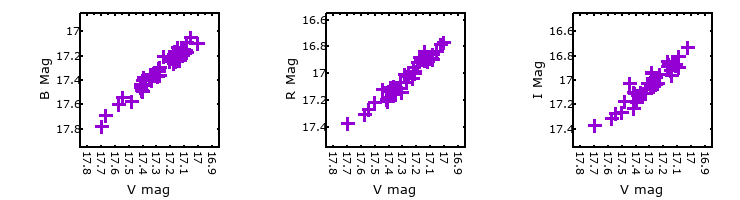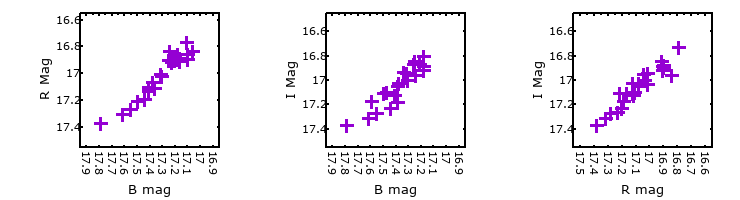### I & R Statistics

Welch & Stetson (1993, AJ, 105, 1813) present a method for varible star detection in CCD images from automated surveys. Data from two bands are compared and two statistics are calculated: R and I.

The I-statistic has an expectation value of zero for stars that are NOT variable. A larger non-zero I-value indicates a higher likelihood that the star is variable. The R statistic indicates how the color of the star changes as the variable grows brighter with R = 1.0 being no change in color.

R and I values have only been calculated if there are more than four (4) matched measurements of brightness in each band.
V
(I|R)
B
(I|R)
R
(I|R)
B
(I|R)
16.87 | 0.78
R
(I|R)
15.64 | 1.3710.85 | 0.63
I
(I|R)
10.41 | 1.688.89 | 1.167.93 | 1.44